Density Estimation edit page

In many cases texture measurements are acquired in the form of a series of points or intensities. EBSD measurements are usually a grid of measurement points, while pole figure measurements are often angular positions combined with intensity values. However, in many cases we want to do analysis that requires a continuous function, in which case we want to determine the continuous function that best represents our data points. This section discusses the mathematical basis of this calculation and how it is affected by some of the parameters involved.

In mathematical terms, density estimation is a concept that describes estimation of a probability density function $$f_N$$ from given random samples $$x_n$$, $$n=1,\ldots,N$$. In the simplest case the random samples $$x_n$$ are real numbers and come from an unknown distribution function $$f$$. The goal is to ensure that $$f_N$$ approximates $$f$$ as well as possible.

Lets illustrate this starting with the example of a mixed Gaussian distribution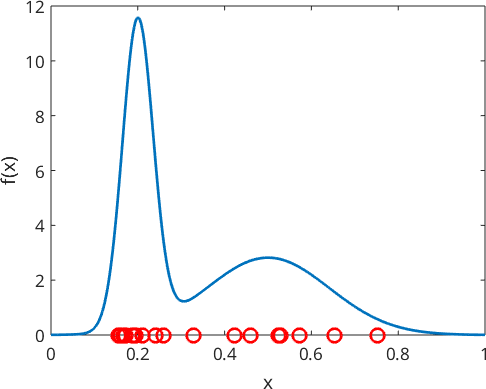Note that the higher the peak of the original function, the more points randomly generated. Because the red points are randomly generated, your plot will look slightly different.

## The Histogram

The easiest way to estimate a density function from the sample $$x_n$$ is with a histogram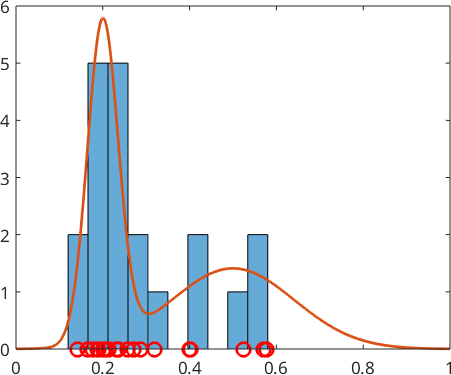However, since the histogram always leads to a piecewise constant function (step function) the fit to the true density function $$f$$ is usually not so good. A better alternative is kernel density estimation.

## Kernel Density Estimation

The idea of kernel density estimation is to pick some kernel function $$\psi$$, e.g. a Gaussian with mean $$0$$ and stadard deviation $$0.05$$,

shift its center to the position of each sample points $$x_n$$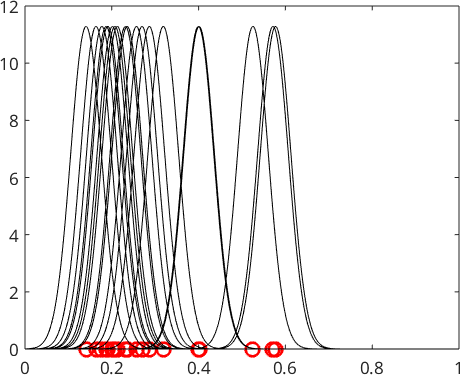and take the mean

$f(x) = \frac{1}{N} \sum_{n=1}^N \psi(x-x_n)$

of all the these shifted kernel functions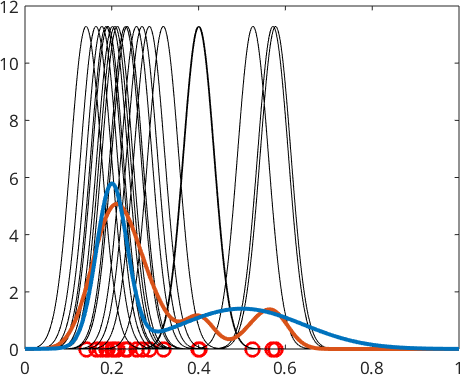We observe that this gives a much better approximation to true density function $$f$$. The most important parameter when computing the kernel density estimate of a random sample is the halfwidth or standard deviation of the corresponding kernel function. Lets repeat the above density estimation with three different standard deviations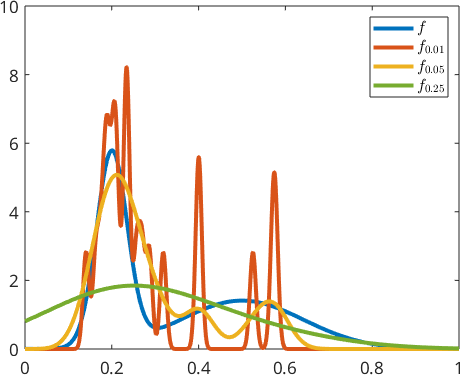In general a too small halfwidth leads to heavily oscillating functions, while a too large halfwdith will result in excessively smooth functions. In the case of one dimensional data kernel density estimation MTEX includes automatic optomization of the halfwidth when using the command calcDensity.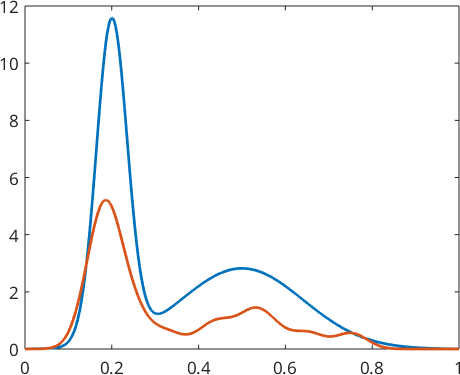## Optimal Halfwidth Selection

Selecting an optimal kernel halfwidth is a tough problem. MTEX provides a couple of methods for this purpose which are explained in detail in the section Optimal Kernel Selection.

## Kernel Density Estimation in d-Dimensions

The command calcDensity may also be applied to $$d$$-dimenional data. For simplicity lets consider a two-dimensional example where both $$x$$ and $$y$$ coordinates are distributed according to the distribution $$f$$ defined at the very beginning of this section.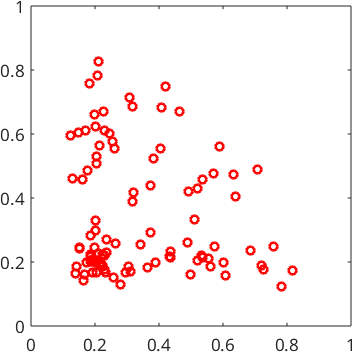Similarly to the one dimensional example we need to specify the range of the $$x$$ and $$y$$ coordinates for the estimated density function. The format is [xMin yMin; xMax yMax].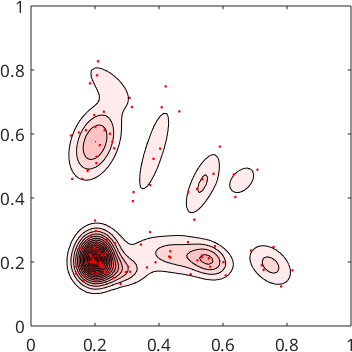## Density Estimation for Directional Data

Kernel density for directional (misorientation/ crystallographic axis) data works analogously as for real valued data. Again we have to choose a kernel function $$\psi$$ with a certain halfwidth $$\delta$$. Than the kernel functions are centered at each direction of our random sampling and summed up. Lets us demonstrate this procedure for misorientation axes between two phases in an EBSD map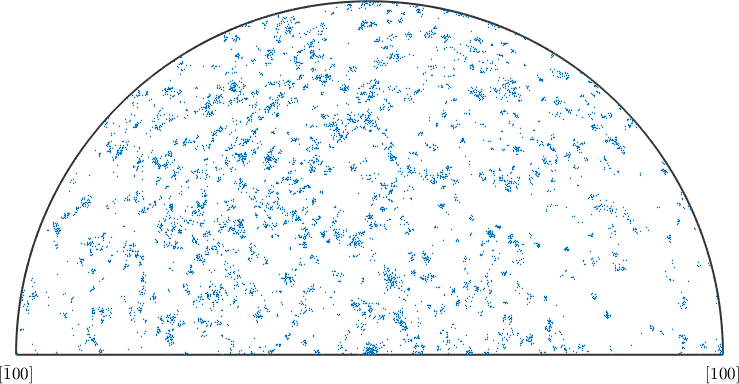The distribution of the misorientation axes may be analyzed in more detail by computing the misorientation axis distribution function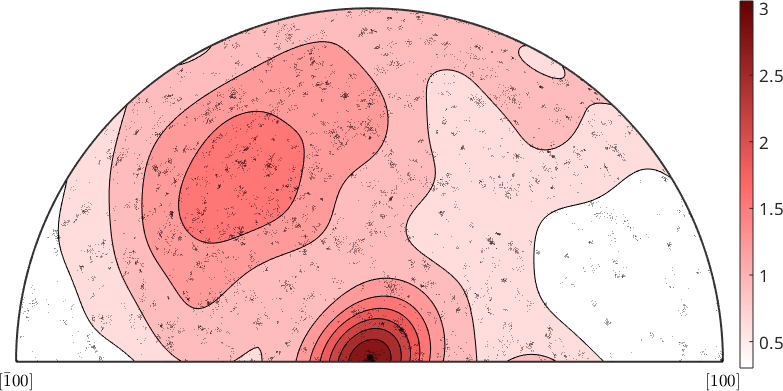Note that the resulting variable axisDensity is of type S2FunHarmonicSym and allows for all the operations as explained in the section Operations on Spherical Functions. In order to stress once again the importance of the choice of the halfwidth of the kernel function we perform the same calculation as above but with the halfwidth set to 5 degree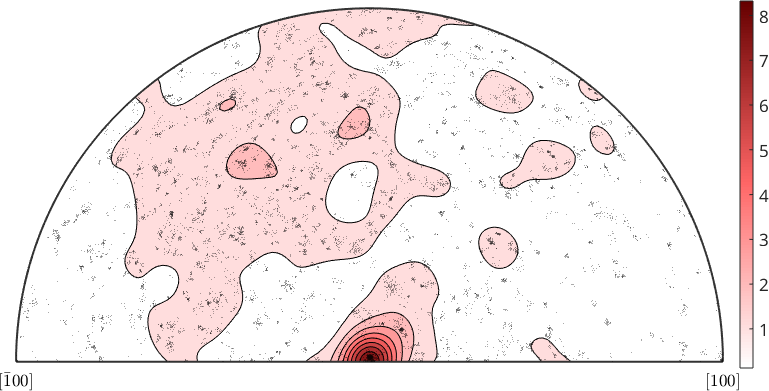## Density Estimation for Orientation Data

Density estimation from orientations sets the connection between individal crystal orientations, as e.g. measured by EBSD, and the orientation distribution function of a specimen. Considering the Forsterite orientations from the above EBSD map the corresponding ODF computes to

Lets visualize the ODF in phi2 sections and plot on top of it the individual orientation measurements from the EBSD map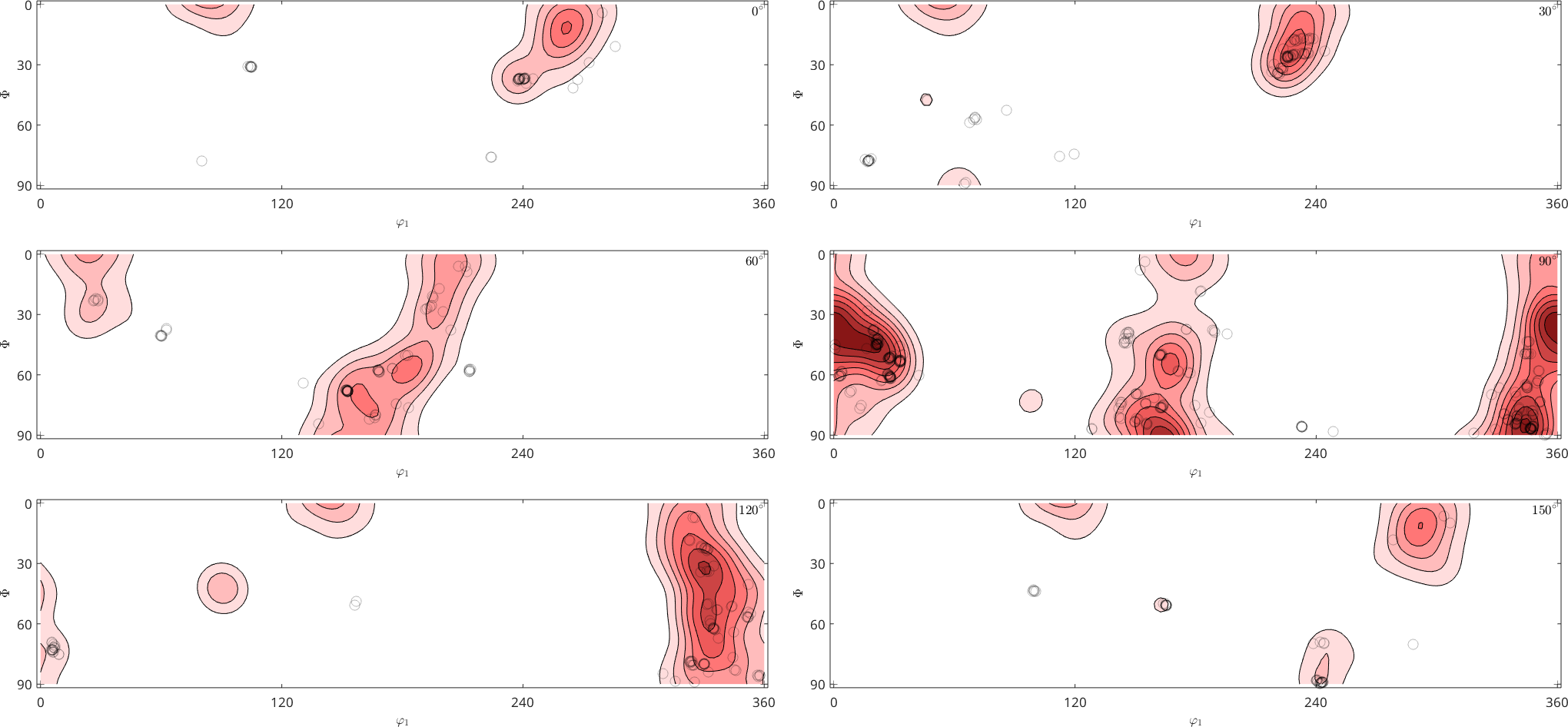A more detailed describtion of ODF estimation from individual orientation measurements can be found in the section ODF Estimation from EBSD data.

## Parametric Density Estimation

In contrast to kernel density estimation, parametric density estimation makes the assumption that the true distribution function belongs to a parametric distribution family, e.g. the Gaussian. In this case it estimates the parameters of this distribution from the random sample. In the case of the Gaussian distribution these parameters are the mean value and the standard deviation. On spheres and in orientation space, the analogous functions to the Gaussian are the Bingham distributions. The estimation of Bingham parameters from directional and rotational data is explained in the sections The Spherical Bingham Distribution and The Rotational Bingham Distribution.

## Density Estimtation with Weights

In many use cases one has a weighted random sample. A typical example is if one wants to estimate a orientation distribution function from grain orientations. In this cases big grains should contribute more to the ODF than small grains. For that reason the functions calcDensity allow for an additional option 'weights' which will pass weights to the density estimation.